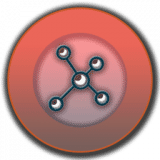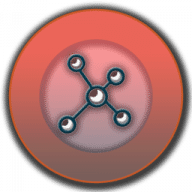Quantum Calculator
1.1

0.0# Quantum Calculator

1.1
11 July 2019

Calculations for quantum physics.

## Overview

Quantum Calculator comprises a selection of 4 different calculators that will simplify calculations which have given you headaches so far. Complex calculations, such as Amplitude Resonance Angular frequency, Ramsauer Townsend Effect, and Step Potential Probability Current for Zone II will be handled easily with this app.

• Amplitude resonance angular frequency
• Ramsauer-Townsend effect
• Step potential probability current for zone II
##### Features
• Formula and description for each calculator
• Copy / Paste params and results
• Clean, simple and easy to use